# 原神璃月地区隐藏怪物类宝箱详解

2022-04-15编辑：jackey

1.传送至望舒客栈传送点，后转身向下滑翔，路过客栈拱门后下落在这堆油纸伞处，走过所有油纸伞后清除掉出现的史莱姆便会刷新宝箱啦。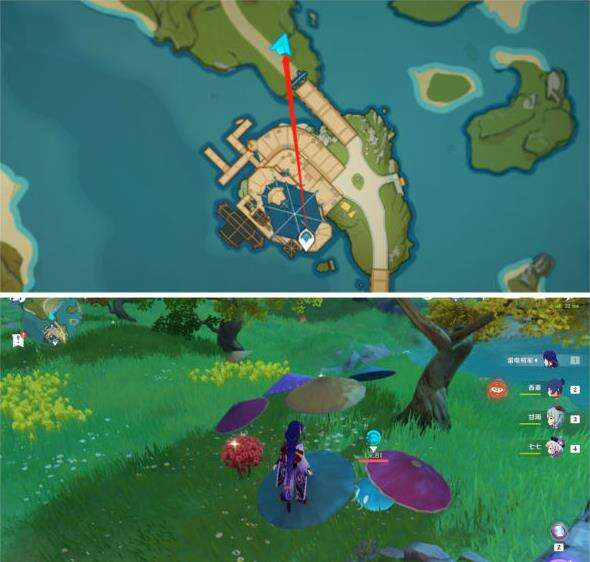2.拿完宝箱1后沿着土路往前，途径下图所示位置会刷新一只火法师，清理掉之后会出现一个珍贵宝箱。

（如果是已经领取过了这里就不会再刷新法师。）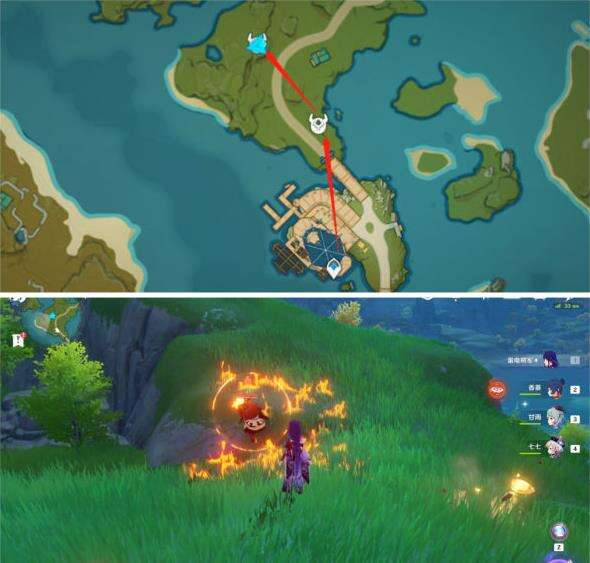3.传送至望舒客栈左上角传送点，左转后直行来到此处，去到下图所示三块巨石中间后会刷新冰、火、雷三只史莱姆，清理完后获得一个精致宝箱。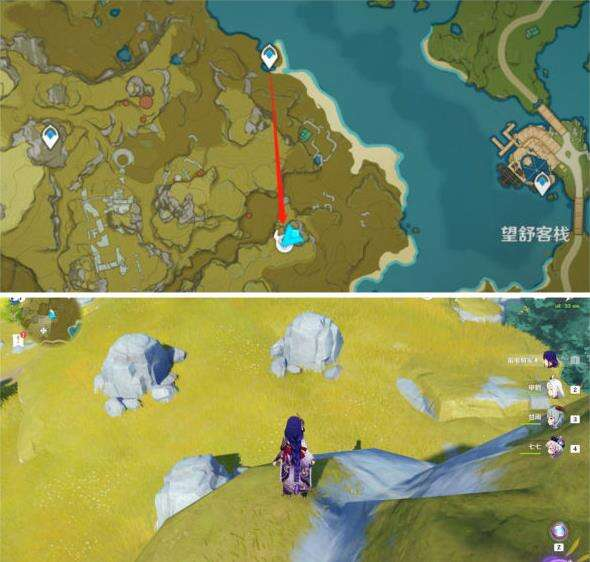4.传送至翠玦坡上方传送点，右转沿着土路往上，到达上方竹篱旁会刷新一只岩史莱姆，清理后获得一个普通宝箱。5.传送至孤云阁秘境处，右转直行来到下图所示小岛，清理掉出现的三只丘丘人便会刷新宝箱。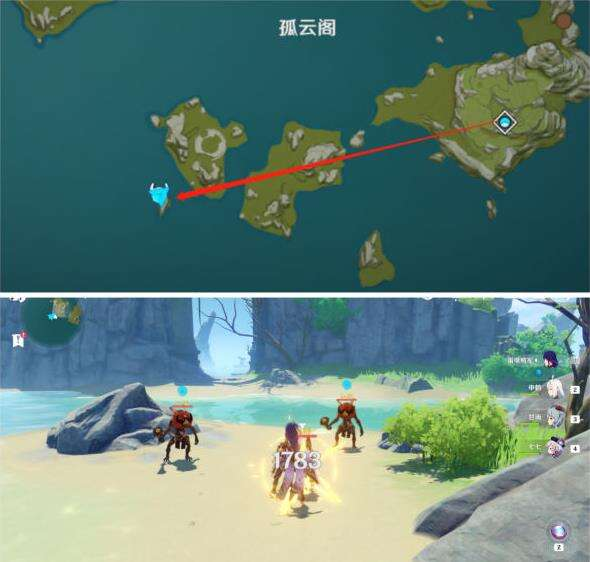​​​​​​​​​​​​​​​​​​​​​​​​​​​​​​​​​​​​​​​​​​​​​​​​​​​​​​​​​​​​​​​​​​​​​​​​​​​​​​​​​​​​​​​​​​​​​​​​​​​​​​​​​​​​​​​​​​​​​​​​​​​​​​​​​​​​​​​​​​​​​​​​​​​​​​​​​​​​​​​​​​​​​​​​​​​​​​​​​​​​​​​​​​​​​​​​​​​​​​​​​​​​​​​​​​​​​​​​​​​​​​​​​​​​​​​​​​​​​​​​​​​​​​​​​​​​​​​​​​​​​​​​​​​​​​​​​​​​​​​​​​​​​​​​​​​​​​​​​​​​​​​​​​​​​​​​​​​​​​​​​​​​​​​​​​​​​​​​​​​​​​​​​​​​​​​​​​​​​​​​​​​​​​​​​​​​​​​​​​​​​​​​​​​​​​​​​​​​​​​​​​​​​​​​​​​​​​​​​​​​​​​​​​​​​​​​​​​​​​​​​​​​​​​​​​​​​​​​​​​​​​​​​​​​​​​​​​​​​​​​​​​​​​​​​​​​​​​​​​​​​​​​​​​​​​​​​​​​​​​​​​​​​​​​​​​​​​​​​​​​​​​​​​​​​​​​​​​​​​​​​​​​​​​​​​​​​​​​​​​​​​​​​​​​​​​​​​​​​​​​​​​​​​​​​​​​​​​​​​​​​​​​​​​​​​​​​​​​​​​​​​​​​​​​​​​​​​​​​​​​​​​​​​​​​​​​​​​​​​​​​​​​​​​​​​​​​​​​​​​​​​​​​​​​​​​​​​​​​​​​​​​​​​​​​​​​​​​​​​​​​​​​​​​​​​​​​​​​​​​​​​​​​​​​​​​​​​​​​​​​​​​​​​​​​​​​​​​​​​​​​​​​​​​​​​​​​​​​​​​​​​​​​​​​​​​​​​​​​​​​​​​​​​​​​​​​​​​​​​​​​​​​​​​​以上就是原神璃月地区隐藏怪物类宝箱详解的全部内容了，希望对大家有帮助。​​​​​​​

#### 相关阅读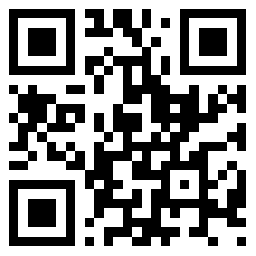• 新闻
• 攻略
• 下载
• 专区